###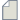Author Topic: Curie temperature  (Read 91 times)

0 Members and 1 Guest are viewing this topic.

####Chem_Prakash

• New ATK user
•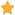• Posts: 2
• Country: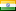• Reputation: 0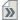##### Curie temperature
« on: April 22, 2022, 09:27 »
I want calculate Curie temperature of a magnetic material using QuantumATK 2018.06. I have tried for the calculation as described in tutorial using the given script. But the calculation ended with an attribute error. The script and the log file are attached herewith, please help me with correct script for my version of ATK. Thanks in advance

Scrpirt:

import pylab

filename = 'B2_7GNR_dos_HE.hdf5'

# Calculate the Curie temperature within the mean field approximation (MFA).
T_C_MFA = heisenberg_exchange.curieTemperature()

# Calculate the Curie temperature within the random phase approximation (RPA)
# using default q-grid (not converged).
T_C_RPA = heisenberg_exchange.curieTemperatureRPA()

# Setup a list with number of q-points in each direction.
number_of_qpoints = numpy.array([10, 20, 30, 40])
T_C_RPA_q_grid = []

# Calculate the Curie temperature for increasing grid sizes.
for nq in number_of_qpoints:
q_grid = MonkhorstPackGrid(nq, nq, nq)
T_C_RPA_q_grid.append(heisenberg_exchange.curieTemperatureRPA(q_grid=q_grid).inUnitsOf(Kelvin))

# Make a linear fit to 1/nq vs. T_C
fit = numpy.polyfit(1/number_of_qpoints, T_C_RPA_q_grid, 1)

# Evaluate the fit at 1/nq = 0 corresponding to infinite number of q-points.
T_C_RPA_extrapolate = numpy.polyval(fit, 0) * Kelvin

# Print the results
nlprint('-------------------------------------------------')
nlprint('Curie temperature:')
nlprint('-------------------------------------------------')
nlprint('MFA                                  : {: .1f}'.format(T_C_MFA))
nlprint('RPA (default q-grid)                 : {: .1f}'.format(T_C_RPA))
nlprint('RPA (extrapolate to infinite q-grid) : {: .1f}'.format(T_C_RPA_extrapolate))
nlprint('-------------------------------------------------')

# Plot the data and linear fit
pylab.figure()
pylab.plot(1/number_of_qpoints, T_C_RPA_q_grid, 'ro', label='Data')
pylab.plot([0, 0.12], numpy.polyval(fit, [0, 0.12]), 'k-', label='Fit')
pylab.xlabel('\$1/n_q\$')
pylab.ylabel('Curie temperature (K)')
pylab.legend(loc=0)
pylab.show()

log file:

+------------------------------------------------------------------------------+
|                                                                              |
| QuantumATK 2018.06-SP1-1[Build 3f8f0aa]                                      |
|                                                                              |
+------------------------------------------------------------------------------+
Traceback (most recent call last):
File "curie_temperature.py", line 9, in <module>
T_C_MFA = heisenberg_exchange.curieTemperature()
AttributeError: 'HeisenbergExchange' object has no attribute 'curieTemperature'

####filipr

• QuantumATK Staff
• Regular ATK user
•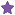• Posts: 27
• Country:• Reputation: 3
• QuantumATK developer##### Re: Curie temperature
« Reply #1 on: April 22, 2022, 15:49 »
The 'curieTemperature()' function was first introduced in version 2021.06, so I highly recommend you to upgrade.

You can also try to implement it yourself given the formula as documented here